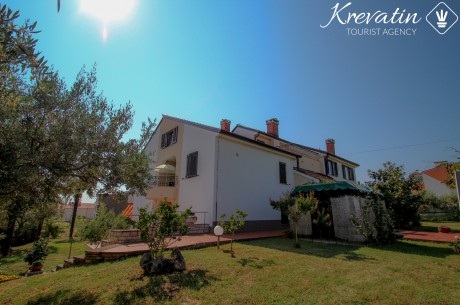• Zambratija
• 300m
•200m
• 4500m

•  a4+2

Sluki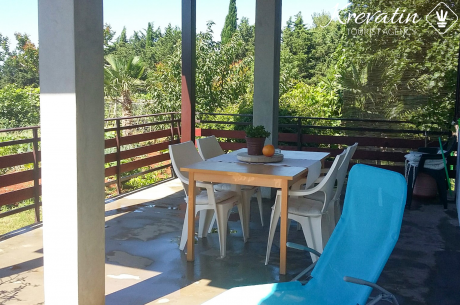• 150m
•200m
• 1000m

•  a2+2

Dario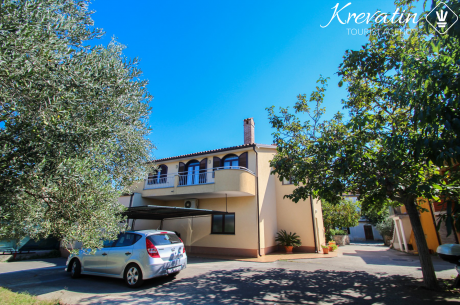• 70m
•200m
• 400m

•  a2

•  a2

Murve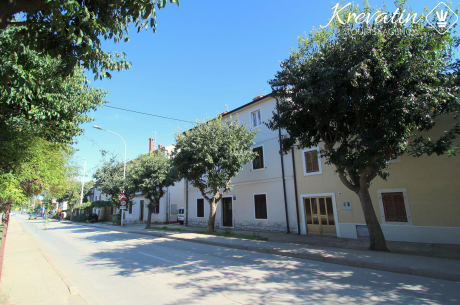• 80m
•200m
• 400m

•  a4+1

Lana• 5m
•200m
• 400m

•  a2+1

Ikac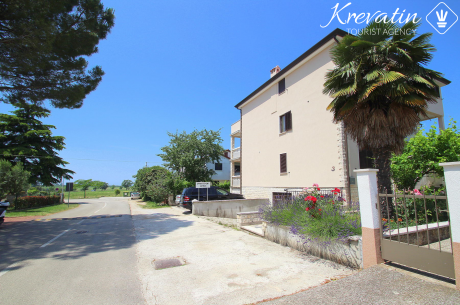• 250m
•250m
• 1500m

•  a4

Rigo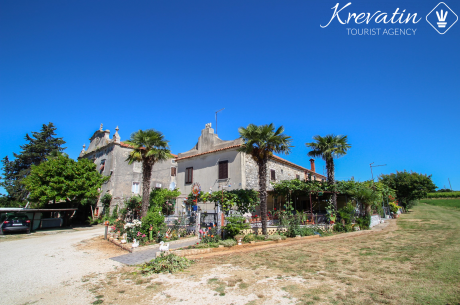• 250m
•250m
• 1500m

•  a2+2

•  a4+2

•  a3+2

•  a2+1

Doris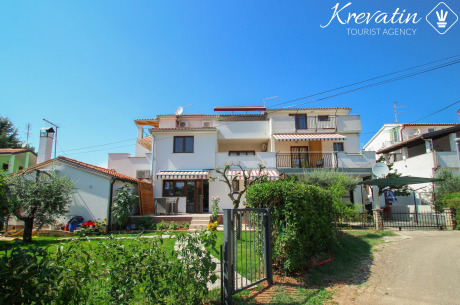• 250m
•250m
• 1500m

•  a4

•  a4

Lency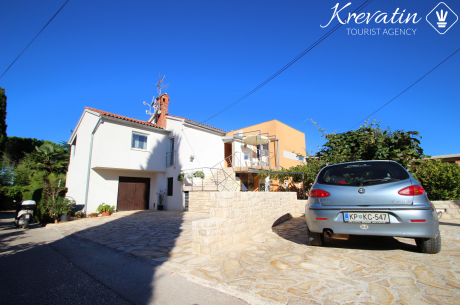• 100m
•250m
• 1200m

•  a2+1

Vesna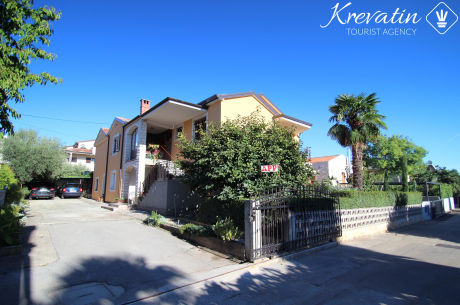• 250m
•250m
• 1500m

•  a2

•  a2+2